# Tips To Prepare Motion In A Straight Line For NSEP

###### ByRamraj Saini###### Synopsis

The chapter on Motion along a straight line is the basics of the Mechanics unit of Class 11 NCERT Physics. Mechanics is an important portion for the National Standard Examination In Physics(NSEP). Read on to know the syllabus and tips to prepare Motion along a straight line for NSEP.###### Synopsis

The chapter on Motion along a straight line is the basics of the Mechanics unit of Class 11 NCERT Physics. Mechanics is an important portion for the National Standard Examination In Physics(NSEP). Read on to know the syllabus and tips to prepare Motion along a straight line for NSEP.

The Class 11 NCERT Physics chapter Motion Along A Straight Line discusses position, frame of reference, basics terms like rest and motion, distance and displacement, speed and velocity, acceleration, uniform and non uniform motion and equations of motion. All these topics are the very basics for mechanics. Questions from the chapter Motion Along A Straight Line are asked in the NSEP exam. In the past six years 1.25 per cent of NSEP questions are from this chapter. Let us have a look at syllabus, questions and reference materials for preparation of Motion Along A Straight Line For NSEP.

Also, Read| How To Prepare NSEP For Ray Optics

## Tips To Prepare Motion Along A Straight Line For NSEP

• Understand the concept of frame of reference

• Study the difference between distance and displacement and speed and velocity

• Study all the graphs and derivations

• Learn the use of equations of uniformly accelerated motion

v=u+at

s=ut+0.5at2

v2=u2+2as

Here u is the initial velocity, v the final velocity and a is the acceleration. Note that the above three equations are only applicable for uniformly accelerated motion.

• Solve problems on free falling

• Solve all the questions from NCERT Class 11 chapter Motion In A Straight Line

• Solve all the NCERT exemplar problems

• Practice NSEP previous year papers

## NSEP Syllabus For Motion In A Straight Line

The following topics are included in NSEP syllabus for Motion In A Straight Line-

Frame of reference, Position-time graph, speed, velocity, basic concepts of differentiation and integration for describing motion, average speed and instantaneous velocity, uniform and non- uniform motion, uniformly accelerated motion, velocity - time and position-time graphs. Equations for uniformly accelerated motion (graphical treatment).

Q-1- The position vector of a point mass is expressed as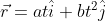The trajectory of the particle is(NSEP 2018)

(a) a straight line. (b) a parabola. (c) a hyperbola. (d) none of the above.

Q-2-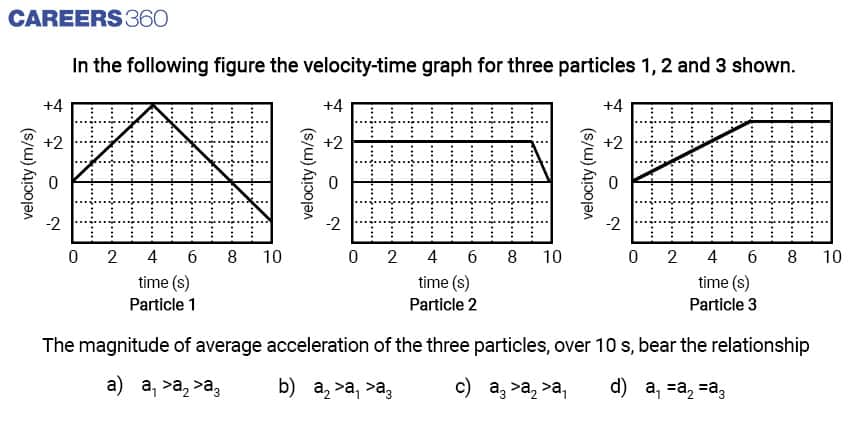## NSEP Study Materials For Motion In A Straight Line

• NCERT Class 11 Chapter Motion In A Straight Line

• NCERT Exemplar Problems For Motion Along A Straight Line

• NSEP previous year question papers

• Physical sciences
• Physics
• Geometry
• Applied and interdisciplinary physics
• Physical quantities
• Classical mechanics
• Mechanical engineering
• Mechanics
• Theoretical physics
• Physical phenomena

## Careers360 helping shape your Career for a better tomorrow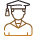#### 250M+

Students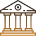#### 30,000+

Colleges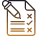#### 500+

Exams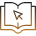E-Books